Next: 4.3 The Pythagorean Theorem Up: 4. Analytic Geometry Previous: 4.1 Addition of Points   Index

# 4.2 Reflections, Rotations and Translations

4.9   Definition (Reflections and Rotations.) We now define a family of functions from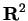to. If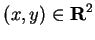, we define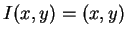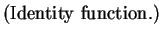(4.10)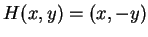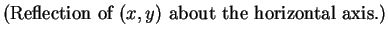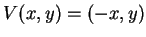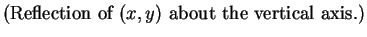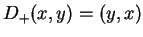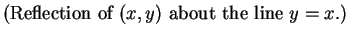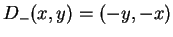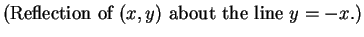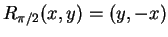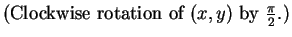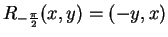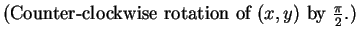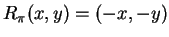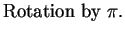(4.11)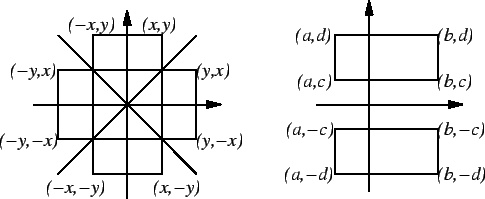Each of the eight functions just defined carries every box to another box with the same area. You should be able to see from the picture that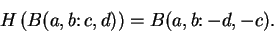We can see this analytically as follows: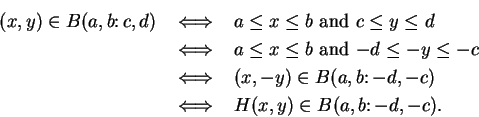I will usually omit the analytic justification in cases like this.

Each of the eight functions described in definition 4.9 carries the square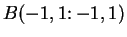to itself.

4.12   Definition (Symmetry of the square.) The eight functions defined in equations (4.10)-(4.11) are called symmetries of the square.

4.13   Exercise. Let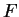be the set shown in the figure. On one set of axes draw the sets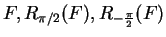and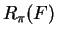(label the four sets). On another set of axes draw and label the sets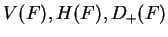and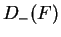.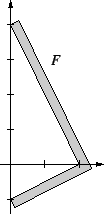4.14   Example. Let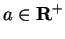and let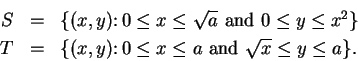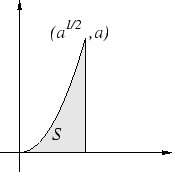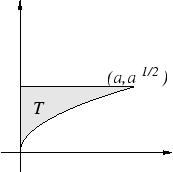From the picture it is clear that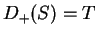. An analytic proof of this result is as follows: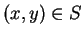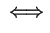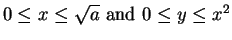(4.15)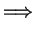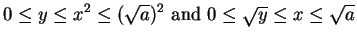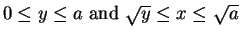(4.16)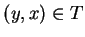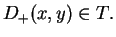To show that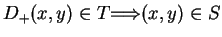, I need to show that (4.16) implies (4.15). This follows because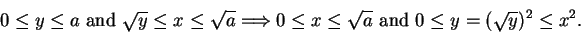In exercise 2.18 you assumed that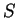and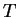have the same area. In general we will assume that ifis a set andis a symmetry of the square, thenand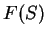have the same area. (Cf. Assumption 5.11.)

4.17   Definition (Translate of a set.) Letbe a set inand let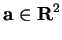. We define the set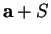by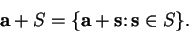Sets of the formwill be called translates of.

4.18   Example. The pictures below show some examples of translates. Intuitively each translate ofhas the same shape asand each translate ofhas the same area as.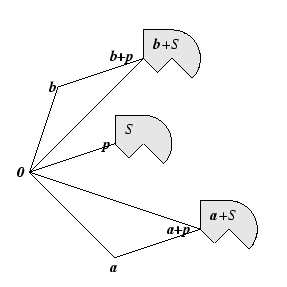4.19   Example (Translates of line segments.) Let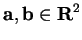. If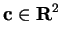, then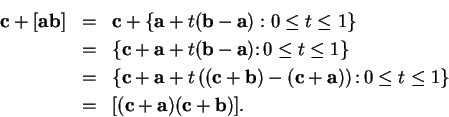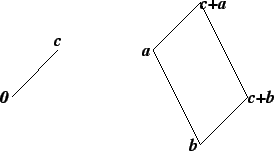In particular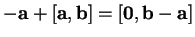, so any segment can be translated to a segment with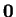as an endpoint.

4.20   Exercise. Let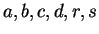be real numbers with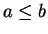and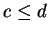. Show that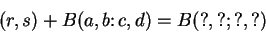if the four question marks are replaced by suitable expressions. Include some explanation for your answer.

4.21   Exercise. Let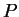be the set shown in the figure below.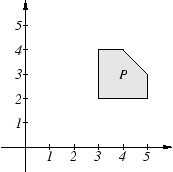a) Sketch the sets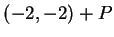and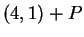.

b) Sketch the sets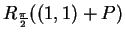and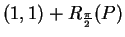, where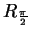is defined as in definition 4.9Next: 4.3 The Pythagorean Theorem Up: 4. Analytic Geometry Previous: 4.1 Addition of Points   Index
Ray Mayer 2007-09-07